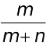# Important Terminology

## Random Experiment

Experiments in which total number of possible outcomes are known in advance but occurrence of some specific outcome cannot be predicted before completion of the experiment is called a random experiment. The probability deals in evaluating the probable occurrence or non-occurrence of different outcomes of random experiments.

Examples of random experiments: Tossing of a coin, throwing of a die, drawing of cards from a well shuffled deck of cards, tossing of two coins etc.

### Sample Space

The collection of all the possible outcomes of a random experiment is called sample space of the experiment and is denoted by S, each member of the sample space is called a sample point or a case.

For example in throwing two coins sample space is {HH, HT, TH, TT}.

### Event

A result of an experiment expressible by a subset of its sample space is called an event of the experiment. An event is denoted mostly by a capital letter A, B, C etc. Each subset of a sample space is an event.

In throwing of two coins some of the events are

A → {HH} {In throwing of two coins exactly two head appears or no tail appears}

B → {HH, HT, TH} {In throwing of two coins atleast one head appears}

C → {HH, HT, TH, TT} {In throwing of two coins at most two head appears}

D→ {HH, TT} {In throwing of two coins same denominations appear on both coins}

### Null Event

An event, which can never occur, is called null or impossible event. It being an empty set is denoted by Φ.

### Sure Event

An event, which is sure to occur on every performance of the experiment, is called sure event or certain event.

### Equilikely Events

Events A, B, C, … are called equilikely if on repeating the experiment a large number of times, there is no reason to expect any one of these more often than the others.

In other words every event of the system has the same expectation to occur.

### Mutually Exclusive Events

Two events A and B are called mutually exclusive if the occurrence of any one precludes the occurrence of the other. i.e In any performance of the experiment the two events can not occur together. I.e. A ∩ B = Φ.

Events A1, A2, … An are called mutually exclusive if the occurrence of any one of these precludes the occurrence of at least one of the others. I.e., A1 ∩ A2 ∩ … ∩ An = Φ.

Pair - Wise Mutually Exclusive Events

Events A1, A2, …, An are called pair-wise mutually exclusive if these are mutually exclusive in pairs. i.e. Ai ∩ Aj = Φ for all i ≠ j.

NOTE A system of pair-wise mutually exclusive events is always mutually exclusive. The converse may not be true.

### Exhaustive System of Events

A system of events is said to be exhaustive if on each performance of the experiment at least one of the events of the system is must to occur.

E.g. (i) On tossing of a coin, the events {H} and {T} form an exhaustive system of events.

(ii) On throwing of a die the events {1, 2}, {2, 3, 4}, {5} and {4, 5, 6} form an exhaustive system.

### Odds in Favour and Odds Against an Event

In a random experiment if there are total m + n mutually exclusive, exhaustive and equally likely sample points (cases) and m cases are in favour of an event and n cases are against it than m : n is called odds in favour of event A and n : m is called odds against it.

Probability of event A, P(A) =.